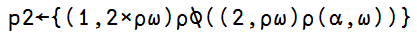# More fun with APL

Note: see the rest of the series so far.

I stumbled across this post the other day and problem 2 seems like something I can easily do in APL since it essentially requires you to interleave two arrays.

The problem is:

Write a function that combines two lists by alternatingly taking elements. For example: given the two lists [a, b, c] and [1, 2, 3], the function should return [a, 1, b, 2, c, 3].

Here’s the solution I have come up with:since it uses both $latex \omega$ (right argument) and $latex \alpha$ (left argument) so it’s a dyadic function, let’s test it out:

$latex ‘a’ \ ‘b’ \ ‘c’ \ p2 \ 1 \ 2 \ 3$

=> a 1 b 2 c 3

Here’s how it works:

• concatenate the two arguments together, with the left argument first $latex (\alpha, \omega)$
• reshape $latex \rho$ the concatenated vector into 2 rows, so that you have effectively placed $latex \alpha$ and $latex \omega$ into a matrix, i.e.

$latex a \ b \ c\\* 1 \ 2 \ 3$

• transpose that matrix

$latex a \ 1\\* b \ 2\\* c \ 3$

• reshape $latex \rho$ the transposed matrix into a vector, and that’s it!

### 7 thoughts on “More fun with APL”

1.Assuming that you have only vectors, my solution to this would be ,?,??.

2.Strictly speaking your result is a one row matrix rather than a vector. If it’s truly a vector that you want, then your code can be simplified by using ravel rather than reshape (and some of the parentheses can be removed too):

s1?{,?(2,??)??,?}

These days I prefer to use the monadic function Tally (?) rather than monadic ?: Tally returns the length of the leading axis, which in the case of a vector is its length. Tally has the benefit of always returning a scalar.

s2?{,?(2,??)??,?}

A more traditional solution would be to use Catenate (,) with fractional axis:

s3?{,?,[?io+.5]?}

although unless you use the slightly awkward ?io+.5 the expression is ?IO dependent.

Recent versions of Dyalog include Table (?) which has been in Sharp APL and J for many years. Using Table allows the following solution:

s4?{,?,??}

The last expression is the fastest, but as to which is “best” .. well that is in the eye of the beholder !

3.that’s amazing ;-)

4.Thanks for these, that’s one of the things I like above APL – there’s always so many different ways to approach a given problem!

5.Thanks Fiona :-)

6.Nechemyeh Ben Tzien Brudzewsky

Now that we have function trains, I like:
s5?,,[1.5]